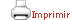Teaching plan for the course unit

(Short version)

 Castellano English CloseGeneral information

Course unit name: Nonlinear Programming and Flows in Networks

Course unit code: 361227

Coordinator: Julia De Frutos Cachorro

Department: Department of Economic, Financial and Actuarial Mathematics

Credits: 6

Single program: S

 Estimated learning time Total number of hours 150

 Face-to-face and/or online activities 60
 -  Lecture with practical component Face-to-face and online 30 -  IT-based class Face-to-face and online 30
 Supervised project 40
 Independent learning 50

 Learning objectives
 Referring to knowledge The course is based on the study and solving of decision problems using techniques that allow the systematic identification and evaluation of all possible decision options associated with the problem. Moreover, whenever the nature of the problem so allows, these problems are best formulated in mathematical terms. The modeling of problems using nonlinear programming (NLP) provides a closer perspective of the reality studied.The aim of the course as regards NLP, therefore, is to be able to determine the optimal decision of a problem;in addition, when a problem is subject to constraints, it is useful to know whether or not the problem has a solution and, if so, what its location and nature are. In the case of network flows, given that many of these problems can be solved by linear programming, the aim of the course is to present the basic notions of this theory and to recognize different types of network flow problems and to study specific algorithms with which they can be solved.  Referring to abilities, skills In the case of nonlinear programming (NLP), students should be able to: — Formalize simple situations using NLP. — Apply the concepts of NLP to find results and deduce their nature. — Interpret the results obtained in the context of the model. — Analyse economic models from the point of view of NLP. — Apply NLP to optimization problems in statistics. — Solve problems by applying the techniques studied and using computer programs, such as SAS and Excel. In the case of network flows, students should be able to: — Formulate problems using linear programs. — Distinguish the peculiarities of different types of network. — Formalize simple situations such as network flows, identifying the elements and procedures for solving them. — Apply different types of algorithm in the same network model and compare their results — Solve problems by applying the techniques studied. — Interpret the results and develop a critical capacity. — Define and solve network flow problems through the use of computer programs, such as SAS and Excel.

 Teaching blocks

1. Nonlinear programming

1.1. Introduction to nonlinear programming: preliminary concepts and definitions

1.2. Solving nonlinear programming problems with no constraints

1.3. Nonlinear programming methods based on approximations

1.4. Nonlinear programming with constraints: Method of Lagrange multipliers and Kuhn-Tucker conditions

1.5. Algorithms for solving nonlinear problems with constraints

1.6. Applications

2. Network flows

2.1. Network models: basic definitions and examples

2.2. Shortest path problem

2.3. Maximum flow problem

2.4. Minimum-cost flow problem

2.5. Other applications

Book

AHUJA, Ravindra K. et al. Network Flows. Theory, algorithms, and applications. Upper Saddle River (N.J.): Prentice Hall, 1993

BAZARAA, Mokhtar S. et al . Linear programming and network flows. Hoboken, N.J.: John Wiley & Sons, 2010

BALBÁS, Alejandro. et al. Programación Matemática. 2a ed. Madrid: AC, 1990

MARTÍN, Quintín. et al. Investigación Operativa. Problemas y ejercicios resueltos. Madrid [etc.]: Pearson Educación, 2005

SYDSÆTER, Knut. et al., Further Mathematics for Economic Analysis. 2nd edition, Finantial Times/Prentice Hall, 2008.

TAHA, Hamdy A. Investigación de operaciones. 9a ed. México [etc.]: Pearson Educación, 2012

WINSTON, Wayne L. Investigación de operaciones. Aplicaciones y algoritmos. México: Thomson, 2005